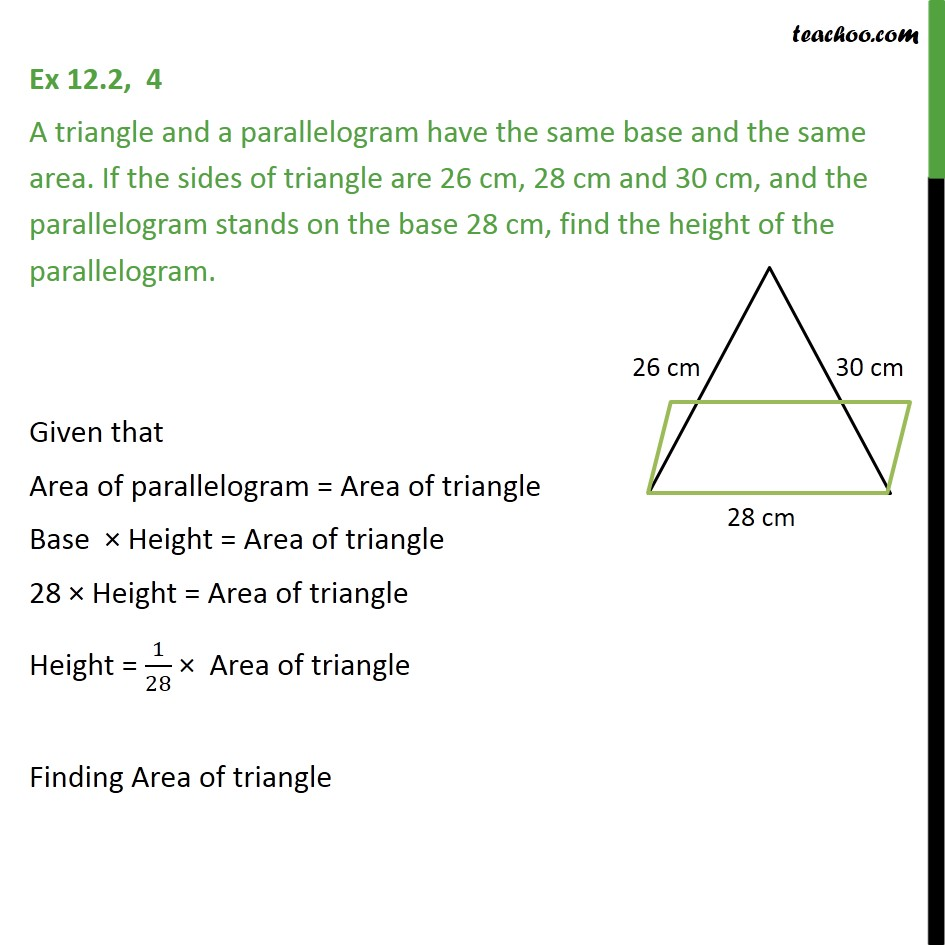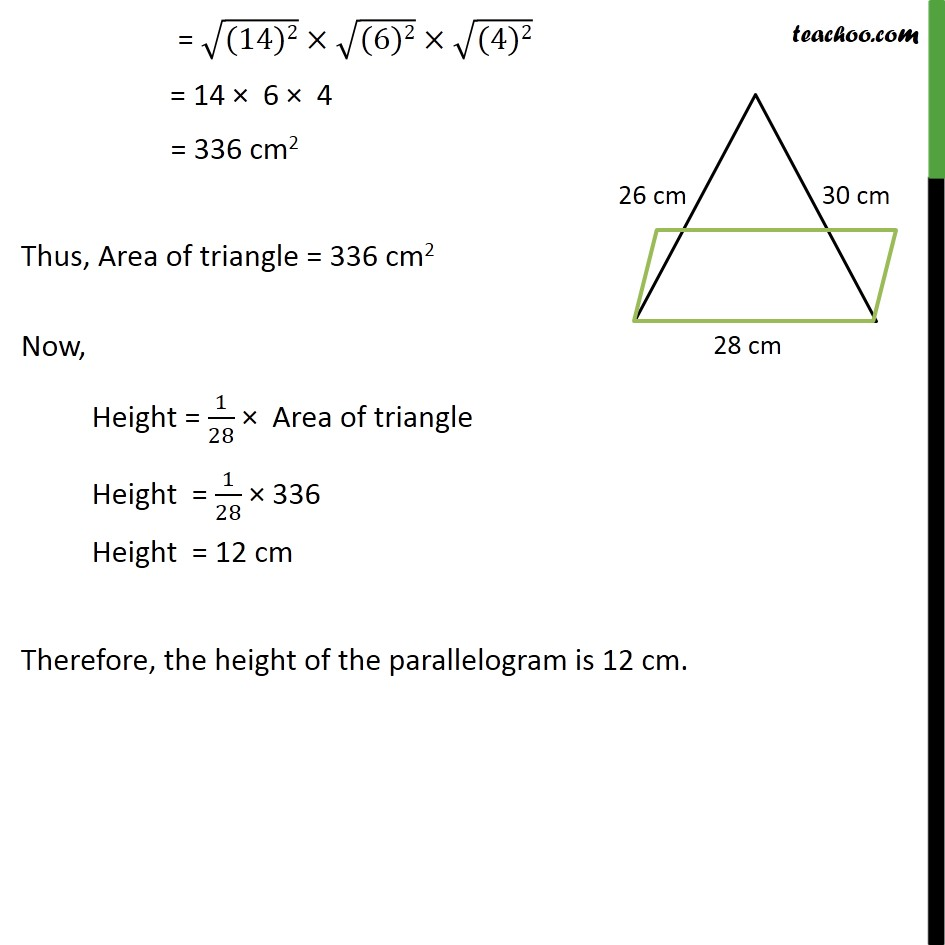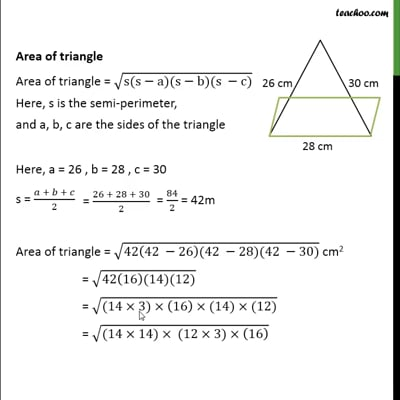Ex 12.2

Chapter 12 Class 9 Herons Formula
Serial order wiseThis video is only available for Teachoo black users

Maths Crash Course - Live lectures + all videos + Real time Doubt solving!

### Transcript

Ex 12.2, 4 A triangle and a parallelogram have the same base and the same area. If the sides of triangle are 26 cm, 28 cm and 30 cm, and the parallelogram stands on the base 28 cm, find the height of the parallelogram. Given that Area of parallelogram = Area of triangle Base Height = Area of triangle 28 Height = Area of triangle Height = 1/28 Area of triangle Finding Area of triangle Area of triangle Area of triangle = (s(s a)(s b)(s c)) Here, s is the semi-perimeter, and a, b, c are the sides of the triangle Here, a = 26 , b = 28 , c = 30 s = ( + + )/2 Area of triangle = (42(42 26)(42 28)(42 30)) cm2 = (42(16)(14)(12)) = ((14 3) (16) (14) (12)) = ((14 14) (12 3) (16) ) = ((142) (36) (16) ) = ((142) (62) (42) ) = ((14)2) ((6)2) ((4)2) = 14 6 4 = 336 cm2 Thus, Area of triangle = 336 cm2 Now, Height = 1/28 Area of triangle Height = 1/28 336 Height = 12 cm Therefore, the height of the parallelogram is 12 cm.